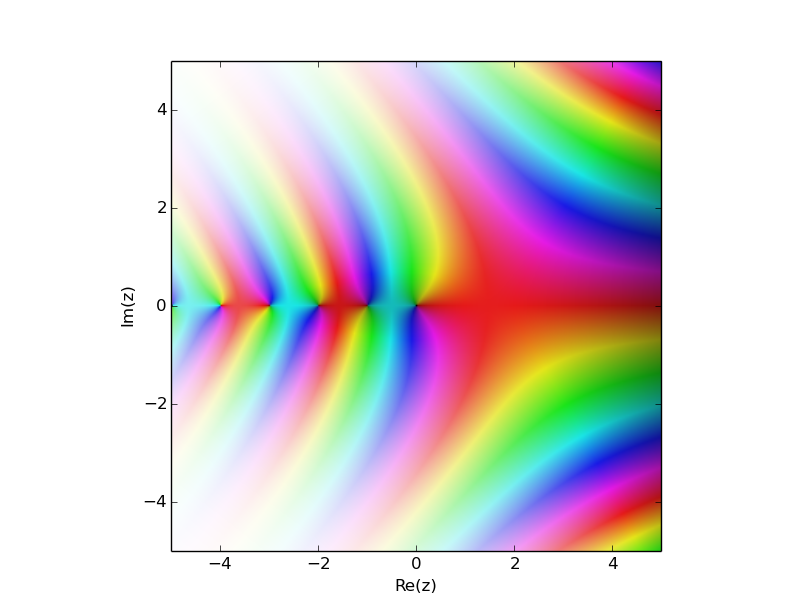# Arb and FLINT in Python

January 7, 2015

My programming project over the holidays was to resurrect the Python bindings for FLINT that I originally wrote in 2012. Among other enhancements, Python-FLINT now wraps Arb for real and complex numbers, polynomials and matrices. I'm already having a lot of fun being able to work interactively with Arb in Python!

>>> from flint import *
>>> ctx.pretty = True; ctx.unicode = True  # tweak output format
>>> ctx.dps = 50
>>> arb.pi()
[3.1415926535897932384626433832795028841971693993751 ± 6.84e-51]


Take a moment to appreciate the beautiful output. The interval-to-string conversion took some time to design (it is currently only part of the Python interface, not the C library). When printing a real or complex number, you always get a decimal representation that is guaranteed to be correct up to an error of at most one unit in the last digit. For added clarity, an explicit radius is printed by default. In fact, the output can be parsed back from a string to an interval, guaranteed to contain the original (in general, you get a slightly larger interval due to the roundings both ways).

The following happens when the result only is accurate to 10 digits, or no digits at all:

>>> (arb.pi() + arb(1)/10**40).sin()
[-1.00000000e-40 ± 1.96e-50]
>>> arb.pi().sin()
[± 9.70e-51]


The tentatively named function good takes a function, evaluates it, and automatically repeats with increased precision as necessary until it has a result accurate to the current working precision (or a number of digits that can be passed as input). Here we compute an accurate value of $\operatorname{erf}(100) - 1$, which involves more than 4000 digits of cancellation (of course, there should be an erfc function for this, but it illustrates the principle):

>>> good(lambda: acb(100).erf() - 1, maxprec=10**6)
[-6.405961424921732039021339e-4346 ± 1.49e-4371]


Complex numbers and various transcendental functions work. This computes $\zeta(0.5+14.1i)$:

>>> ctx.dps = 30
>>> acb("0.5","14.1").zeta()
[0.0046984001834891354049188962 ± 4.14e-29] + [-0.027058282374250772881980468 ± 4.19e-28]j


You can do easy plotting via mpmath:

>>> from mpmath import fp
>>> fp.cplot(lambda z: acb(z).rgamma(), points=1e5)Functions are implemented as methods, not as global functions (though global functions might appear in a later version). Clearly distinguishing between the implementations for different types can be useful. For example, you can use a method on the fmpq type to compute a Bernoulli number as an exact rational number, and a method on the arb type to compute it as a real number:

>>> fmpq.bernoulli_ui(100)  # exact
-94598037819122125295227433069493721872702841533066936133385696204311395415197247711/33330
>>> arb.bernoulli_ui(100)   # approximate
[-2.838224957069370695926416e+78 ± 4.56e+53]
>>> arb.bernoulli_ui(10**19)   # too big for fmpq!
[-1.093349247859552504076114e+177675256497386331238 ± 1.13e+177675256497386331213]


Polynomial arithmetic works. For example, we can construct the rising factorial $(x)_n = x (x+1) \cdots (x+n-1)$ with $n = 9$ as a polynomial, using repeated multiplication. Or to be fancy, we can construct the same polynomial by evaluating the rising factorial function at 10 arbitrarily chosen complex numbers and doing polynomial interpolation. Note the error bounds!

>>> ctx.dps = 15
>>> reduce((lambda x, y: x*y), [acb_poly([k,1]) for k in range(9)])
1.00000000000000*x^9 + 36.0000000000000*x^8 + 546.000000000000*x^7 + 4536.00000000000*x^6 + 22449.0000000000*x^5 + 67284.0000000000*x^4 + 118124.000000000*x^3 + 109584.000000000*x^2 + 40320.0000000000*x
>>> xs = [acb(1+1j)**k for k in range(10)]
>>> ys = [x.rising_ui(9) for x in xs]
>>> acb_poly.interpolate(xs, ys)
([1.0000000000000 ± 4.53e-15] + [± 3.15e-15]j)*x^9 + ([36.000000000000 ± 2.37e-13] + [± 2.32e-13]j)*x^8 + ([546.0000000000 ± 5.45e-12] + [± 4.80e-12]j)*x^7 + ([4536.000000000 ± 5.89e-11] + [± 6.25e-11]j)*x^6 + ([22449.00000000 ± 3.96e-10] + [± 3.98e-10]j)*x^5 + ([67284.00000000 ± 1.65e-9] + [± 1.82e-9]j)*x^4 + ([118124.000000 ± 5.27e-9] + [± 5.09e-9]j)*x^3 + ([109584.000000 ± 9.28e-9] + [± 9.12e-9]j)*x^2 + ([40320.0000000 ± 9.45e-9] + [± 9.67e-9]j)*x + ([± 3.35e-9] + [± 3.72e-9]j)


We can also use a product tree to construct a polynomial quickly from its roots. The following computes an approximation of the central coefficient in the polynomial $(x)_{10000}$, i.e. the Stirling number $S_1(10000,5000)$. This takes 1.5 seconds (computing the exact value using fmpz_poly arithmetic takes 25 seconds):

>>> n = 10000; f = arb_poly.from_roots(range(-n+1,1)); print f[n//2]
[9.05633652381e+21281 ± 5.66e+21269]


There's rudimentary code for matrices. The following evaluates $\exp(A) \exp(-A) = I$ with $A = \left(\begin{smallmatrix}1 & 2 \\ 3 & 4\end{smallmatrix}\right)$:

>>> A = arb_mat(2,2,[1,2,3,4])
>>> A.exp() * (-A).exp()
[[1.000000000000000000000 ± 6.55e-24],                          [± 3.28e-24]]
[                        [± 1.17e-23], [1.0000000000000000000000 ± 6.50e-24]]


This is just a preview. I have a lot more to fix before announcing a release worthy of a version number.

FLINT and Arb are huge libraries (comprising over 5000 C functions), and Python-FLINT currently only exposes a bare minimum of features. Many more methods are to be added. I haven't added any types for finite fields, $p$-adic numbers, and power series yet. Power series are particularly important for numerics, as they permit rigorously isolating roots, computing integrals (etc.) of analytic functions.

The wrapper code itself is an embarrassment in its current state. Type conversions and coercions are done in a dozen different half-broken ways. But it shouldn't be too hard to clean everything up. In the mean time, feel free to checkout the git repository and play around with it at your own peril.

Python-FLINT is written in Cython. I considered rewriting it from scratch as a pure Python library using ctypes, but this made fmpz(2) + fmpz(2) over ten times slower than the Cython version. Granted, the Cython version is already ten times slower than a raw call to fmpz_add in C due to the cost of creating and destroying Python objects (plus general interpreter overhead), but there's no need to make things even worse!